Thu, Sep 29, 2022 @ 09:00 GMT
HomeContributorsTechnical AnalysisTechnical Outlook and Review

# Technical Outlook and Review

USD/JPY:

On the H4 chart, price has confirmed a bullish momentum breaking the previous high. We are bullish bias- Price has tested the first resistance and pulled back slightly. If bullish momentum continues, it should bring price to 144.906 where the 161.8% extension sits. Alternatively, if price reverses, it might pull back to test the first support at 141.652 where the 23.6% retracement sits then the second support at 139.349 where the 38.2% retracement and overlapping support sits

Areas of consideration:

• H4 time frame, 1st resistance at 144.906
• H4 time frame, 1st support at 141.652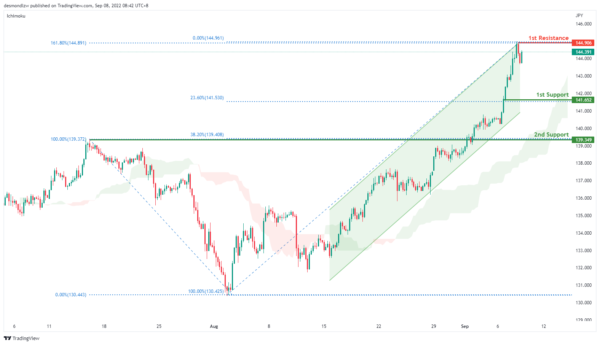DXY:

On the H4, prices have broken the range and are moving in an ascending trend, we are bullish bias. Price has pulled back slightly but if bullish momentum continues, it should bring price up to 110.582 where the 100% projection sits and subsequently the second resistance at 111.045 where the 138.2% fibonacci extension sits. Alternatively, price might pull back to test the first support at 109.258 where the 23.6% retracement and previous swing high sits and subsequently the second support at 108.106 where the 61.8% projection sits

Areas of consideration:

• H4 time frame, 1st resistance at 110.582
• H4 time frame, 1st support at 109.258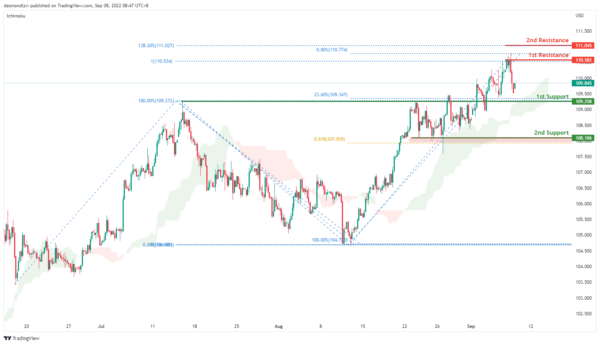EUR/USD:

On the H4, price has broken the range and is moving in a descending trend – we are bearish bias. Price has pulled back slightly but if bearish momentum continues, it should test the intermediate support at 0.9868 then the first support at 0.9802 where the 61.8% projection sits. Alternatively if price rebounds, it might test the first resistance at 1.0118 where the 50% retracement and 61.8% projection sits and then the second resistance at 1.02745 where the 78.6% retracement and 100% projection sits.

Areas of consideration :

• H4 1st resistance at 1.0118
• H4 1st support at 0.9868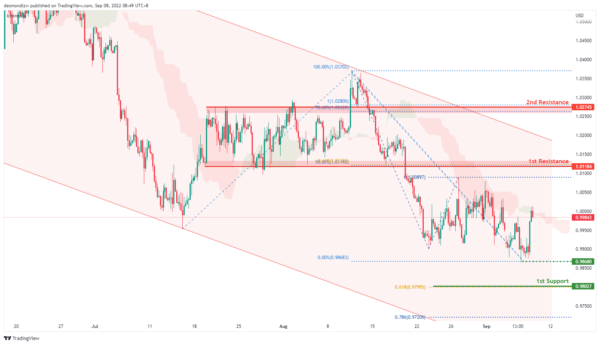GBP/USD:

On the H4, prices seem to still be in a bearish momentum and respecting the ichimoku cloud. Prices have rebounded slightly but if bearish momentum continues it should move toward the first support at 1.1437 levels where the 161.8% extension sits. Alternatively, if it fails to break this level, it might look to test the first resistance at 1.1613 where the previous swing high sits subsequently the second resistance at 1.1760 level where the 61.8% projection and 38.2% retracement sits

Areas of consideration:

• H4 1st resistance at 1.163
• H4 1st support at 1.1437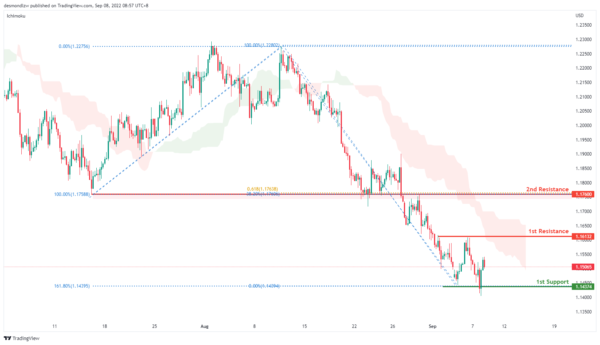USD/CHF:

On the H4, prices have rejected the 0.9859 levels and we are currently bearish bias. Price has bounced off the first resistance at 0.9859 where the previous swing high sits. If price do not break the first resistance, it could pull back to test the first support around the 0.9742 levels where the 23.6% retracement and swing low sits, subsequently the second support at 0.9626 where the 50% fibonacci retracement,78.6% projection and previous swing low sits

Areas of consideration

• H4 1st support at 0.9742
• H4 1st resistance at 0.9859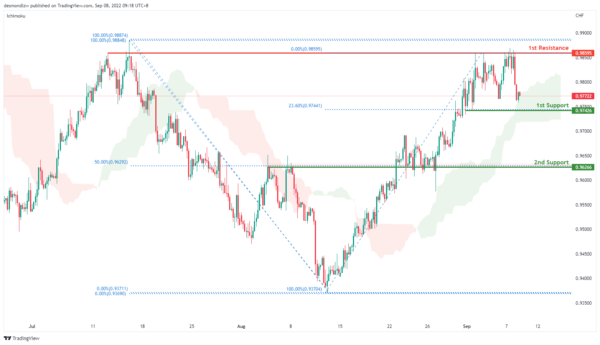XAU/USD (GOLD):

On the H4, with prices below ichimoku cloud and descending trendline, we have a bearish bias. The 1st support level could be at 1689.529, which is in line with the previous swing low and 100% fibonacci projection. If this support level is broken, the 2nd support level is at 1680.546, where the previous significant swing low and 61.8% fibonacci projection are. Alternatively, the price may continue the pullback trend and break the descending trendline. The key resistance level could be at 1730.482 area, which is in line with the overlap resistance, 50 % and 38% fibonacci retracement, as well as 100% fibonacci projection.

Areas of consideration:

• H4 time frame, 1st support at 1689.529
• H4 time frame, 2nd support at 1680.546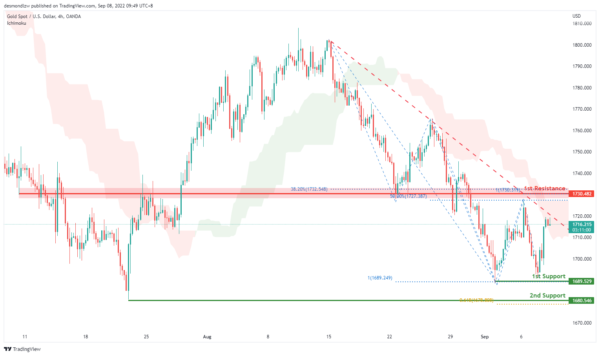AUD/USD:

On the H4, with the price moving below the ichimoku cloud and moving within the descending channel, we have a bearish bias that the price may drop to the 1st support at 0.66844, which is in line with the swing low. If the price can break this support level, the 2nd support level could be at 0.66325, where the 78.6% fibonacci projection is. Alternatively, the price may rise to the 1st resistance 0.67770, where the 23.6% fibonacci retracement and overlap resistance are.

Areas of consideration

• H4 1st support at 0.66844
• H4 2nd support at 0.66325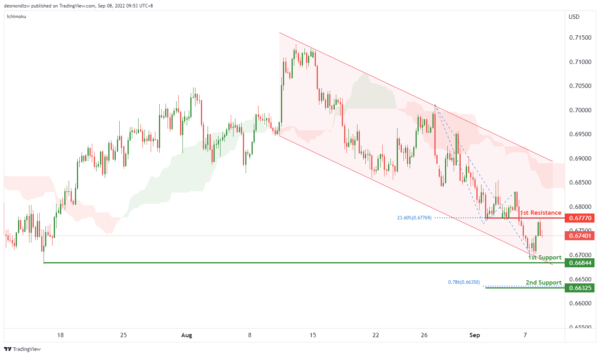NZD/USD:

On the H4, with price moving within the descending channel and below ichimoku indicators, we have a bearish bias that the price may drop to the 1st support at 0.60031, which is in line with the 61.8% and 78.6% fibonacci projection. If the price can break the 1st support, the next support could be at 0.59472, where the 127.2% fibonacci extension and 100% fibonacci projection are. Alternatively, the price may rise to test the 1st resistance at 0.60780 again, where the overlap resistance is. If the price can break the 1st resistance, the 2nd resistance level could be at 0.61264, which is in line with the 50% fibonacci retracement and overlap resistance.

Areas of consideration:

• H4 time frame, 1st support at 0.60031
• H4 time frame, 2nd support at 0.59472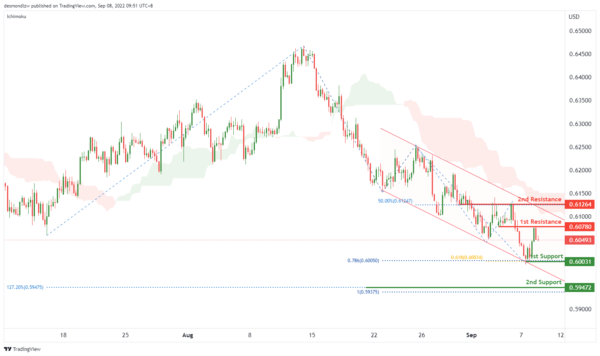On the H4, with the price moving within the ascending channel, above ichimoku cloud, we have a bullish bias that the price may rise from the 1st resistance at 1.31922, where the swing high is to the 2nd resistance at 1.32236, where the significant swing high and 100% fibonacci projection are. Alternatively, the price may drop to the 1st support at 1.30988, where the 23.6% fibonacci retracement and previous swing lows are.

Areas of consideration:

• H4 time frame, 1st resistance at 1.31922
• H4 time frame, 2nd resistance at 1.32236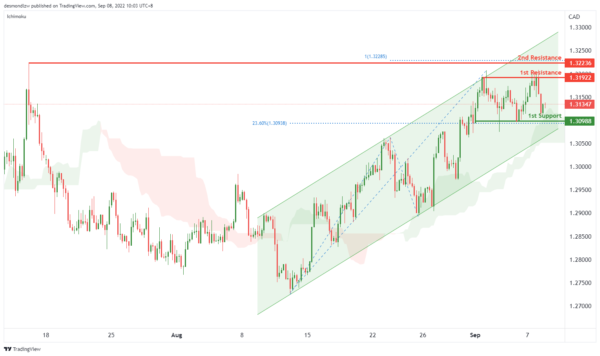OIL:

On the H4, with price below ichimoku cloud and below the descending trendline, we have a bearish bias that the price may drop to the 1st support at 88.332, where the 78.6% fibonacci projection is. If the price could break the 1st support, the 2nd support could be at 85.715, where the 100% fibonacci projection is. Alternatively, the price may continue the pullback trend and rise to the 1st resistance at 93.159, where the 50% fibonacci retracement and previous swing support are.

Areas of consideration:

• H4 time frame, 1st support at 88.332
• H4 time frame, 2nd support at 85.715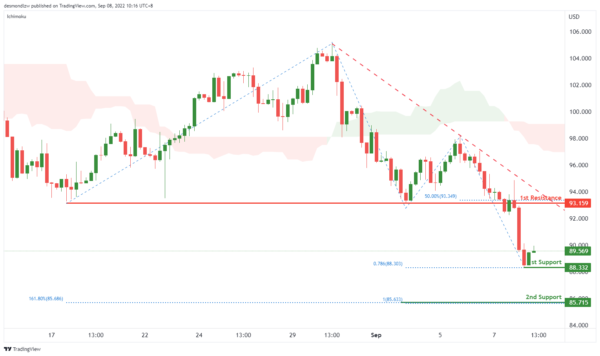Dow Jones Industrial Average:

On the H4, with price moving below the ichimoku indicator, we have a bearish bias that price will drop from 1st resistance at 31904 where the pullback resistance and 23.6% fibonacci retracement are to the 1st support at 31025 where the swing low support is. Alternatively, price could break 1st resistance and rise to 2nd resistance at 32632 where the pullback resistance, 50% fibonacci retracement and 78.6% fibonacci projection are.

Areas of consideration:

• H4 time frame, 1st resistance of 31904
• H4 time frame, 1st support at 31025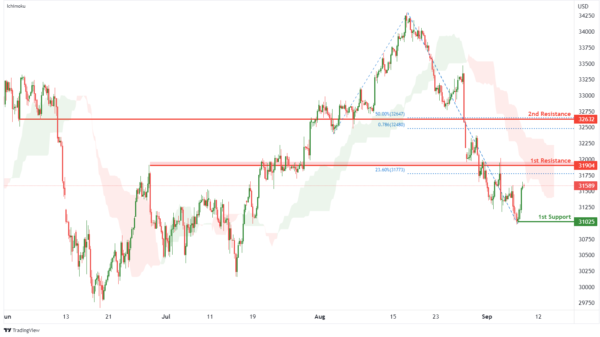DAX:

On the H4, with price moving below the ichimoku indicator, we have a bearish bias that price will drop from 1st resistance at 13017.19 where the pullback resistance is to the 1st support at 12601.62 where the swing low support and 78.6% fibonacci projection are. Alternatively, price could break 1st resistance structure and rise to 2nd resistance at 13361.47 where the overlap resistance, 61.8% fibonacci retracement and 78.6% fibonacci projection are.

Areas of consideration:

• H4 time frame, 1st resistance of 13017.19
• H4 time frame, 1st support at 12601.62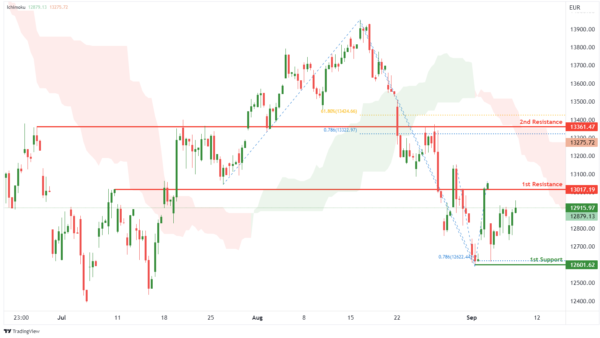ETHUSD:

On the H4, with price moving within an ascending channel and above the ichimoku indicator, we have a bullish bias that price will rise to 1st resistance at 1651.19 where the pullback resistance is. Once there is upside confirmation that price has broken 1st resistance structure, we would expect bullish momentum to carry price to 2nd resistance at 1723.42 where the swing high resistance and 78.6% fibonacci projection are. Alternatively, price could drop to the 1st support at 1562.81 where the overlap support is.

Areas of consideration:

• H4 time frame, 1st resistance of 1651.19
• H4 time frame, 1st support at 1562.81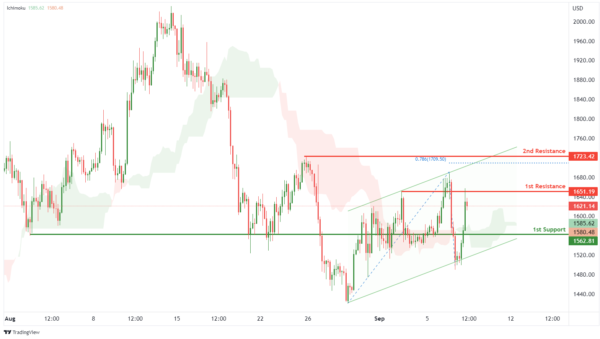BTCUSD:

On the H4, with price moving below an ichimoku indicator, we have a bearish bias that price will drop from the 1st resistance at 19498.02 where the pullback resistance and 23.6% fibonacci retracement are to the 1st support at 18588.89 where the swing low support and 78.6% fibonacci projection are. Alternatively, price could rise to 2nd resistance at 20711.10 where the pullback resistance and 61.8% fibonacci retracement are.

Areas of consideration:

• H4 time frame, 1st resistance of 19498.02
• H4 time frame, 1st support at 18588.89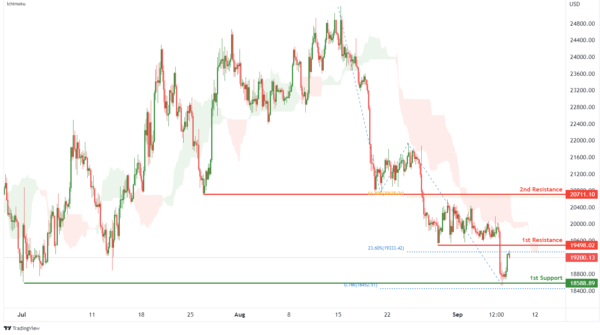S&P 500:

On the H4, with prices breaking out of the ascending trendline and moving below the ichimoku indicator, we have a bearish bias that the price will drop to the 1st support at 3945.01 where the pullback resistance is. Once there is downside confirmation that price has broken 1st support structure, we would expect bearish momentum to carry price to 2nd support at 3722.42 where the swing low support is. Alternatively, price could rise to 1st resistance at 4089.97 where the pullback resistance, 50% fibonacci retracement and 100% fibonacci projection are.

Areas of consideration:

• H4 time frame, 1st resistance of 4089.97
• H4 time frame, 1st support at 3945.01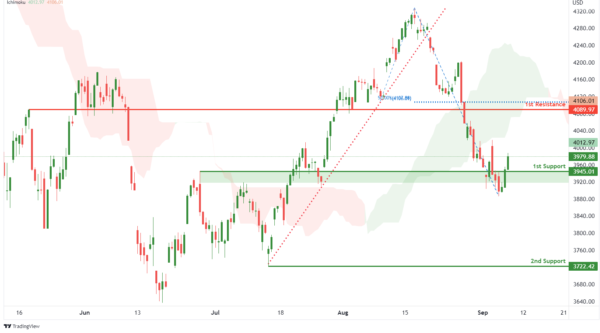IC Marketshttp://www.icmarkets.com/
IC Markets is revolutionizing on-line forex trading; on-line traders are now able to gain access to pricing and liquidity previously only available to investment banks and high net worth individuals.